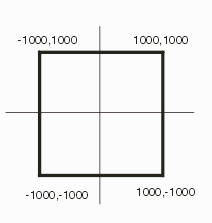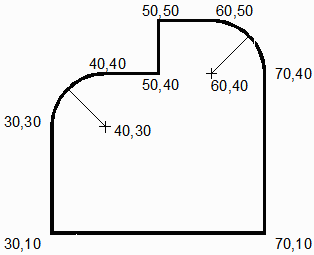### Extrusions

An extrusion consists of one or more 2D closed figures in the xy plane, extruded between two z-values. Any 2D figure after the first one represents a non-touching, non-intersecting hole in the parent figure. The definition is as follows:

`    EXTR <n_children> <z1> <z2>`

Example

`    EXTR 1 100.0 200.0`

An extrusion is defined using the EXTR keyword, followed by the number of 2D figures making up the extrusion and the two z-values between which the figures should be extruded. Three types of figures can be extruded: the 2D polygon, the circle and the path.### 2D Polygon

A 2D polygon is a closed geometry lying in the xy plane, made up of unique points which form non-intersecting straight edges. The definition uses the keyword 2DPG followed by the number of points in the polygon. The points themselves follow, on multiple lines, if required:

```    2DPG <n_points>
<x1> <y1> ... <xn> <yn>
```

Example

```    2DPG 4
1000.0   1000.0
-1000.0   1000.0
-1000.0  -1000.0
1000.0  -1000.0

or

2DPG 4
1000 1000 -1000 1000 -1000 -1000 1000 -1000
```### 2D Polygon Arc

A 2D polygon arc is a closed geometry lying in the xy plane, made up of unique vertices which form non-intersecting straight edges. The connections between vertices can be either lines or arcs. The definition uses the keyword 2DPA followed by the number of vertices in the boundary. The vertex coordinates themselves follow, on multiple lines, if required:```
2DPA <n_segments>
<x1> <y1> <cx1> <cy1> <r1> ... <xn> <yn> <cxn> <cyn> <rn>

```

Example

```2DPA 8
30 10 0 0 0
70 10 0 0 0
70 40 60 40 10
60 50 0 0 0
50 50 0 0 0
50 40 0 0 0
40 40 40 30 10
30 30 0 0 0

```

### 2D Circle

A 2D circle is a circle which lies in the xy plane, with an optional parameter defining the number of chords that may be used when rendering it.

`   2DCR <x> <y> <radius> [<sides>]`

Example

`   2DCR 300.0 400.0 125.0 16`### Path

A PATH is a line with a width defined at each vertex and an endcap definition. Each vertex is defined as an XY pair and line segments connect the vertices. The endcap is optional but is normally assumed to be a half-round in order to be compatible with PCB layout tools.

```PATH <n_points> [<endcap>]
<x1> <y1> <w1> ... <xn> <yn> <wn>```

Example

```   PATH 6 1
```